## General Chemistry

Learn the toughest concepts covered in Chemistry with step-by-step video tutorials and practice problems by world-class tutors

17. Acid and Base Equilibrium

# Identifying Acids and Bases

Acid Identification

Most acids have one common feature and that is the presence of the hydronium ion, which is represented by H+ or H3O+.

1
concept

## Identifying Binary Acids5m
Play a video:

Binary acids can be identified by the fact that they all possess an H+ ion attached to an electronegative element.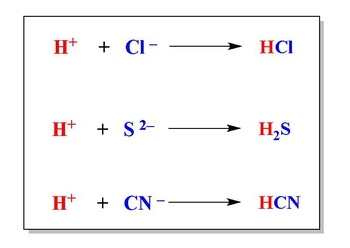Oxyacids can be identified by the fact that they all possess an H+ ion connected to a nonmetal and oxygen, hence the prefix “oxy”.

2
concept

## Identifying Oxyacids2m
Play a video:
Now we're gonna say the second type. They're called oxy acids. And from the name Oxy, you could tell that it has one particular element in their oxygen. So we're gonna say oxy acids are acids that contain H plus I on. Of course, they contain oxygen, and they contained some type of nonmetal. Okay, So the difference between binary and Oxy is that Oxy has oxygen's involved. Binary does not. Something else they both share in common is that they usually do not possess any medals. Again, we're gonna see usually when we have a metal involved, that compound will be a base. Now, we're gonna say that oxy acids are formed when we hydrate non metal oxides. So in this example, here are non metal oxide would be carbon dioxide here and here would be sulfur try oxide. Okay, So non metal oxide just means you have a non metal connected to oxygen. Now all that's gonna happen is the water is going to attach itself to this whole compound. And what we get are two types off oxy acids and their oxy acids. Because both have h both have oxygen. Remember that H is really age plus they both have oxygen, and they both have some type of nonmetal involved. And that's what makes them both oxy acids. So here we would have carbonic acid. And here we have sulfuric acid. And again, we'll learn how to name these particular types of acids later on. But for right now, just realize, how do we create oxy acids? We just add H plus to some type of non metal oxide Co two s +02 s, +03 Those types of compounds, uh, P +03 Those are the kinds of compounds you add water to to make your new types of oxy acids. Now that you guys have seen the two types of assets binary and oxy, I want you guys to try to answer the next following question here. I say which the following compound or compounds cannot be classified as an acid. I gave you just brief description on on both, but try to use your best judgment to determine which one could be in which ones could not be. Then, once you're done with that click on the explanation button, you'll see a video of me explaining how to approach this problem. Guys, good luck

They are created by the hydration of nonmetal oxides.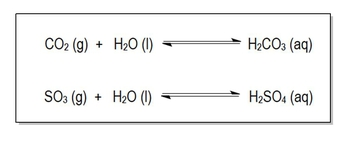Conversely, metal oxides create bases when hydrated by water.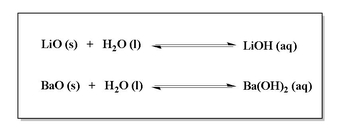3
Problem

Which of the following compound(s) cannot be classified as an acid?

A. H2S

B. HCN

C. H2

D. C2H6

E. All are acids

Binary Acid Strength
4
concept

## Acids and  Electrolytes2m
Play a video:
we talked about there being two classes of assets. There are binary acids and then there are oxy acids for binary acids will go over the different rules that are associated with determining the strengths of different binary acids. Binary acids have their own set of rules. Oxy acids which are a different type of acid will have their own separate list of rules that we follow for them when we're trying to compare the strengths of different oxy acids. Now, regardless if you're looking at a oxy acid or binary acids, we're gonna say strong acids in general are strong electrolytes. So they they ionized completely in water. Here we have hcl which is a strong binary acid. We have a single error going forward to show that it completely ionized it, it goes to completion. So that means we're gonna make 100% of both of these ions, weak acids are weak electrolytes. And again, whether it be weak oxy acids or weak binary acids, weak acids in general are weak electrolytes. So they don't completely ionized in water here, we don't have a solid arrow going forward, we have double arrows to say that instead of going to completion, our reaction reaches an equilibrium. So we're gonna say an equilibrium is established. So that means we're gonna make less than 100% of these ions. And in fact we tend to make a very small amount of these particular ions. So when it comes to weak acids, we're gonna have to basically set up what are called ice charts later on in order to determine the ph of these different types of weak acids. Now, when we're gonna talk about binary acids, keep in mind again, there are two classes of acids. These are just the rules for binary acids. When we talk about oxy acids, they have their own separate list of rules.

Strong Acids are strong electrolytes that completely dissociate into ions when dissolved in water.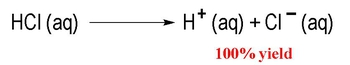Weak acids are weak electrolytes that don’t completely ionize, but instead reach a state of equilibrium.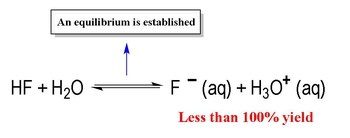5
concept

## Strength of Binary Acids5m
Play a video:
here we can say that the strength of a binary acid is based on the electro negativity, which I'll abbreviate as Ian or size, which is really the atomic radius, which I'll abbreviate as they are. Of the non note. Now, remember we're looking at a periodic table as we head towards the top right corner of the periodic table, the electro negativity will increase and our atomic radius world decrease. Now when it comes to the periodic table, we don't give electro negative values to the noble gasses. Flooring is the most electro negative element on the periodic table, with a value of four point up. Now, for elements in the same period or row, then we look at their electro negativity. We're going to say here, the greater the electro negativity than the more acidic the acid. So let's say for example, I'm comparing CH four, B, H 3 & H. F. So if we look here, B, C and F, they're all in the same roar period with one another. And we would say here the greater the electron activity. The more acidic, boring we just said is the most electro negative element on the periodic table. So a check would be the most acidic out of these three options. Now, if they're in the same in the same group or column, then instead we look at their size, we're gonna say the bigger or the greater greater the atomic radius than the more acidic. So let's say we were comparing hcl hbr and H I. So C A B. R. And I as we go up the group, the atomic radius gets smaller. So you become less acidic i is larger than BR and Cl because it's lowered down the group since it's larger, it's more acidic. So H. I would be the strongest asset out of these three. Alright. So if they're in the same row, we look at electro negativity. If they're in the same group, then we look at size. But let's say they're not in the same group or ro what do we do that? Well, if they're separated by only one row from each other, then we look at their electro negativity. Alright, so how do we do something like that? Let's say we're looking here at Mm Let's say we're looking here at NH three versus H. To us. So N. Is here as is here they're not in the same role when they're not in the same group. We look at electronic activity, nitrogen is more electro negative than sulfur. You don't recall that. Make sure you go back and take a look at my video dealing with the periodic trend of electro negativity. So as a result of this, this should be a little bit more acidic than this. Now, let's say that they're separated by more than one role from each other. If they're separated by more than one role from each other, Then we're going to say here that we look at size. Okay, so let's say we're comparing let's see we're comparing NH three versus H. Two T. They're not separated by one road from each other. This is in row two. This is all the way down here. In row four size is more important, since delirium is lowered down than nitrogen is, it's larger and therefore this would be more acidic. Alright, so just keep these trends in mind when trying to compare different binary acids to one another.

When looking at a binary acid we look at both electronegativity and size to determine their strength.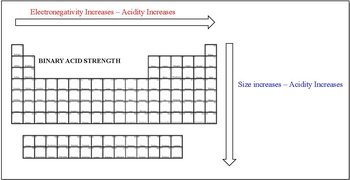6
Problem

Which is the weakest acid from the following?

7
Problem

Which of the following acids would be classified as the strongest?

Oxyacid Strength

The strength of an oxyacid depends on the number of oxygen atoms and the electronegativity of the nonmetal.

8
concept

## The Strength of Oxyacids2m
Play a video:
in this video, we're gonna take a look at Oxy acids strength. So we're gonna say here that the strength of Oxy acids is based on the number off oxygen's or the electro negativity of the nonmetal. We're gonna go over. When do we look at either one? So here we're gonna say the rule is the number one rule for oxy acids is if my oxy acid has two or more oxygen's than hydrogen zones, then my oxy acid is a strong acid. So if we take a look at the first one we have here h n 03 we're gonna say it's an oxy acid because it contains hydrogen, a non metal and an oxygen. Here, the number of oxygen's is three. The number of hydrogen is just one. When we subtract them, we have to oxygen's remaining. You must have a minimum of two oxygen's remaining in order to be considered a strong oxy acid. Here, it meets that requirement, so it's strong. Next one, we have fennel, so C six h five h here it has one oxygen and then it has five plus one more. That's six hydrogen. So in this one, we don't have any oxygen's remaining. Remember, we need a minimum off to oxygen's remaining to be considered a strong oxy acid, so this one doesn't meet the requirements. So it's weak. Then here we have purple MK acid. So here we have four Oxygen's. We have one hydrogen, so we have three oxygen's remaining. So we've met the minimum requirement of having at least two oxygen's left, so this one would be strong. So when it comes to Oxy acids, this is the first rule that you need to remember. You need to have a minimum of at least two more oxygen's than hydrogen to be considered a strong oxy acid. We're gonna move on to the next portion when we talk about this topic of oxy acids. But keep in mind this first important role

If your oxyacid has 2 or more oxygens than hydrogens then your oxyacid is a strong acid.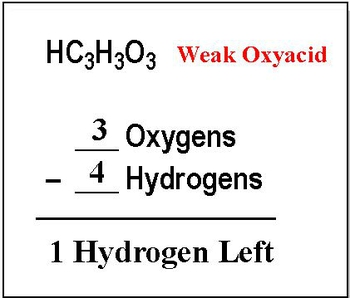9
concept

## Comparing the Strength of Oxyacids3m
Play a video:
when comparing the strengths of different oxy asses remember that if they have a different number of oxygen's than the higher than remaining oxygen's the more acidic. So let's say we're comparing nitric acid versus nitrous acid. This one when we subtract the number of oxygen by hydrogen, we have two oxygen's left. This one would have one oxygen left. So since nitric acid has more remaining oxygen, it's more acidic. But let's say if they have the same number of oxygen remaining, then we'd say the more electro negative, the nonmetal, the more acidic. So let's say for instance, we're looking here at per bronek acid versus same number versus per io take acid. They both have the same job, oxygen's remaining three. So we look at electro negativity which remember as we're heading towards the top right corner of the periodic table will increase grooming is higher up than iodine. So grooming is more electro negative and therefore per bronek acid is more more acidic than per ionic acid. Now, of course, with rules, sometimes you run into exceptions. So here we have three different illustrations here for the first one we have oxalic acid which is H two C 204. When we work out the math, we still have two oxygen's remaining and when we do the same thing with ionic acid, we still have two oxygen remaining, which would tell us it should be a strong they both should be strong oxy acids. But there are exceptions to that rule, the electro negativity of their carbon and iodine is pretty low compared to other non metals. This interferes with their acidity acidity and this is the easiest way to think about this exception because of that oxalic acid and ionic acid are weak oxy acids. Now this last one doesn't necessarily represent only this compound, but it represents a class of compounds it belongs to, which is your alfa terek species. Remember a philatelic species can be acidic or basic for the group of Alphatec species that are not strong oxy acids. Even when following the math, they will have a hydrogen and they will have a negative charge hydrogen because it can be acidic negative charge because they have the ability to be basic. So here doesn't matter that we do the math and it fits the criteria to be a strong oxi acid. It just won't be because it's an esoteric. So, remember our three exceptions are oxalic acid, biotic acid and esoteric species. So just keep these rules in mind when you're trying to compare the strength of different types of oxy acids

When comparing the strengths of different oxyacids remember:

If they have different number of oxygens then the more oxygens the more acidic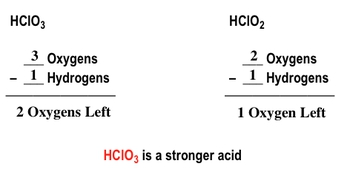If they have the same number of oxygens then the more electronegative the nonmetal the more acidic.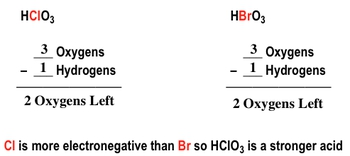With some rules there are exceptions. Oxalic acid and Iodic acid have two more oxygens than hydrogens but are weak acids because carbon are iodine have low electronegativity.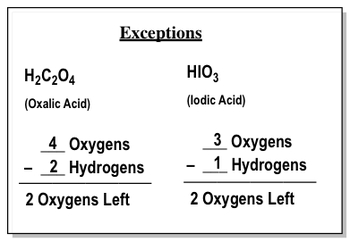Amphoteric species (compounds that can act as both an acid or a base) are also an exception to the rule for oxyacids.

10
Problem

Rank the following oxyacids in terms of increasing acidity.

a) HClO3

b) HBrO4

c) HBrO3

d) HClO4

Base Strength
11
concept

## Bases and Electrolytes2m
Play a video:
Hey, guys, In this new video, we're gonna finally take a look at the strength of bases. So up to this point, we've talked about acids. We've talked about binary acids and oxy acids, and we should know their strengths at this point is, Well, how do we determine when they're weak and what they're when they're strong again? This is important because weak acids, we have to use a nice chart in order to find pH strong acids. We don't have to do that. So what we're gonna say now is let's take a look at bases. We're gonna say strong bases are considered to be strong electrolytes and what a strong electrolytes mean, it means that it completely breaks up or associates when dissolved in water. Here, you're gonna notice that we have a solid era going forward again. This means reaction goes to completion, so all of the reactant will break down basically to 100%. So we get those two ions, we get an A plus O. H minus. Now, weak acids are considered to be weak electrolytes, so they don't completely break down. They don't completely ionized here again will notice that there's a double arrow, meaning that an equilibrium is established. That means our reaction goes in the four direction as well as the reverse direction. Now, Weak acids week faces favor the reactant. All that means is that our reactions really don't break down that much. So a majority of our compound will be in the form of reactant. Very little of it will be made into product in the opposite way. Strong acids and strong bases favor. We're gonna say they favor the products, which means nearly all of our react and breaks down to give us products. So again, remember weak acids week basis. We need to use a nice chart to find pH or P O. H. We'll talk about those later down the road. It's imperative that you guys remember how toe identify things.

Strong Bases are strong electrolytes that completely dissociate into ions when dissolved in water.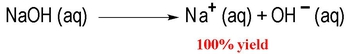Weak bases are weak electrolytes that don’t completely ionize, but instead reach a state of equilibrium.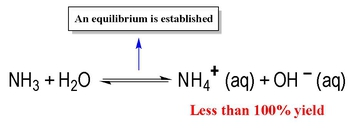Bases are characterized by THREE major features:they may possess metals, they may have a negative charge or they may be an amine

12
concept

## Bases and Group 1A2m
Play a video:
Now, if we take a look here, we're gonna say when it comes to bases, there are three features that were supposed to look out for. Generally, bases possess medals. So from metal is involved, then it's most likely going to be a base. We're going to see a couple exceptions of that when we get to ANFO Terek species. But for right now, let's not worry about that. Next, we're gonna stay basis, tend to have a negative charge. Okay, so bases will have medals or a negative charge, or they'll be classified as another compound, which we call a means now means are or organic type of compound. But we get to see them for the first time in chemistry. So before you guys move onto organic, you get a small taste of what a means are. And how exactly do they relate to bases? So if we take a look at the rules, we're gonna say Group one A. We're gonna say any group won a medal when they combined with any one of these four ions make a strong base. Now, these four ions, we call them hydroxide ion hydrogen can be positive or negative when it's positive it's called Hydro Nia My on. But when it's negative, it's called hydride ion. 02 minuses oxide and NH two minus is a mydd. So notice all of them and with i d e. Because they have a negative charge present. So if one of these negative ion combines with any group won a medal, it's gonna create a strong base. Now recall what are the charges of Group one? A medals group 18 medals are plus one. So, examples we could have any positive ally Positive C s positive K positive. Any of these ions could react with any one of those four ions to give me a strong base. So a year and a positive O H minus combined together to give me an H. This would give me Al I h The two from here would come here. The one from here would come over here CS then here we have K and h two. Remember, you can mix and match any group. When a ion with any one of those four here we get sodium hydroxide, lithium hydride, caesium oxide, potassium hmeid. So, again, any group win a medal with one of those four ions will create a strong base, and strong bases don't require a nice chart in order to find pH or P O. H.

Any Group 1A metal when combined with OH , H , O2– or NH2 makes a STRONG BASE.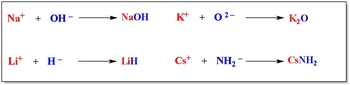13
concept

## Bases and Group 2A2m
Play a video:
next, we're gonna say group to a ions. And here there are There's a caveat. It's not all group to wait. It's certain metals in group two way. So we're gonna say any group to a metal from calcium to bury him when combined with O. H minus, H minus, O to minus or an H two minus will create a strong base. So remember, if you look at your periodic table group two way, we're gonna see beryllium, magnesium, calcium, strontium, barium, and we have our And at the bottom is well, and usually we don't see basis with that, so we're not gonna worry too much about it. So we're saying from calcium and lower. Okay, so the cut off is calcium. These two get left out. So here we're gonna say Group two A is plus two in charge. So we could have these guys reacting with any one of these ions. Okay, so the two from here will come over here. The one from here will move over there cause nothing. So this would be CEO H two here. We're gonna get the to from here. Coming here. B a h two. This is plus two this is minus two. So when they combine, they cancel out, and then the two from here can come down here. So those are all examples of strong bases. Now we're going to stay here, that magnesium is above calcium, and we're gonna say magnesium hydroxide is a base now. Technically, it's a week base. It's not as strong as the others. The others break up 100%. But here's the thing about magnesium hydroxide. We're gonna say a small portion it off. It will dissolve. So let's say about 10% of it dissolves that small portion of it that does dissolve. It dissolves all the way. And when it comes to a weak acids and bases, sometimes a piece of it will dissolve, but not completely for magnesium hydroxide. A small portion of it dissolves and it dissolves completely. Because of that weirdness, we're gonna say M G. O. H. Two is not a strong base, but it's strong enough that we don't need to do a nice chart. So we're gonna say magnesium hydroxide is a weird special case. Technically, it's weak, but it's strong enough that it may not require a nice chart. Just make a little note of that

Group 2A metals, from Ca2+ to Ba2+, when combined with OH , H , O2– or NH2 makes a STRONG BASE.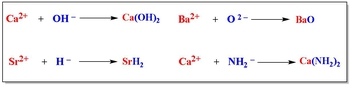Amines are compounds with only nitrogen or hydrogen (i,e. NH3) or with carbon, nitrogen and hydrogen (i,e. CH3NH2).

14
concept

## Amines3m
Play a video:
then finally, the last group we talked about, we're gonna say a means. And since we're gonna meet some room guys to do this, I'm gonna remove myself from the image so we have more room to work with. So it means what the heck isn't a mean what we're gonna say. Inamine is a compound that has, and an H nitrogen and hydrogen such as example, and H three. Or it's a compound that has carbon, hydrogen and nitrogen. Example of that we could have ch three NH two. Okay, so just remember, that means or compounds with nitrogen and hydrogen or carbon, hydrogen and nitrogen. We're gonna say here that neutral a means ones without charges are considered to be weak bases. Examples the two we just wrote and h three or C h three n. H. Two. Now, obviously, there are more than just these, But these air good two examples we have ammonia and then methyl amine methyl amine. The name even tells you that it's in a mean and we're gonna say next that positive, Positively charged. A means are considered to be weak acids. So here we could have NH four positive, which is ammonium. This is ammonia, so ammonium is positive. So it's a weak acid or C H three and H three positive. This would be methyl ammonium. Okay, now we saw compound earlier when we talked about binary acids. That compound was H C N. Now H. C n has the characteristics of on a mean right. I said it was carbon, hydrogen and nitrogen. But remember, you can't be a binary acid and a I mean at the same time, so H. C. And even though it has those three elements is not another mean. It's not in a mean it is a binary acid, so make note of that. This is hydro scion IQ acid, not in a mean, so just make note of that. What in the mean is it usually has just and an H or could have H CNN. HCM, though, is not in a mean it's a binary acid. So look out for those characteristics. And if they give you the name, usually you'll see a meaning the name in some way. That will be a clear giveaway that you're dealing with in a mean now. Hopefully, guys have learned how to identify acids and bases now because again, it's imperative that you know how to identify them as either weak or strong, because if you don't know that, you won't know how to calculate pH or anything else dealing with acid base chemistry.

Neutral Amines are considered weak bases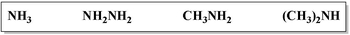Positive Amines are considered weak acids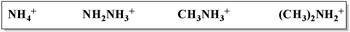15
example

## Classify each of the following as a strong acid, weak acid, strong base or weak base4m
Play a video:
Hey, guys, in this new video, we're gonna put to practice some of the concepts we learned dealing with acids and bases. How doe identify them. And are they weak, or are they strong? So in this first example, it says, classify each of the following as a strong acid, a weak acid, a strong base or a week base. So remember, this is what we're gonna do. This is the protocol to follow when figuring out if something is an acid or base. First, we're gonna ask ourselves some important questions. First, let's identify if it's a base. We're going to say one. Does it have a metal for the first one? It doesn't have a medal to Doesn't have a negative charge anywhere. We don't see a negative charge. Three. Is it gonna mean remember, And a mean has H and N or H C and N doesn't have those lineups, so it's not in a mean. So that means they could not possibly be a base, so that means it's an acid. Is that a binary acid, or is it an oxy acid? If it has oxygen in it, it's a oxy acid, so we know it's not a base. So it has to be an oxy acid because of these oxygen's. We know it's an oxy acid, so let's just do the rules to determine if it's weak or strong. So remember, we look at the number of oxygen's we have to oxygen's minus two hydrogen, leaving us with nothing left. Remember, we need a minimum of two oxygen's left if we don't have that minimum. Couldn't be a strong acid, so this would be a week. Oxy acid. Next, let's go to C. We have H two N N H to ask ourselves the question. Does it have a metal? No. Does it have a negative charge? No. Is it in a mean Remember A means have H and end just like that compound. It has only H and N. Therefore, it isn't a mean. All you say now is Is it positive or is it neutral? It's a neutral, mean and neutral means are weak bases. So this particular week basis called hydrazine to so here. We're going to say this is a week base because it's a neutral amine. Next one be. Does it have a metal? No. Does it have a negative charge? No. Is it in a mean? It has a ches. It has carbon and it has a nitrogen, so it's definitely a mean. Now we just ask ourselves, Is it positive or is it neutral? Since it's positively charged and they will tell you? Since it's positively charged, it's in a mean it's a positive. I mean, so it must be a weak acid. And indeed, the last one. Does it have a medal? No. Does it have a negative charge? No. Is it in a mean? Does it have a Chand N? Nope. Does it have HC N? Nope. So it's an acid. If it has oxygen, it's an oxy acid. Here it has oxygen, so it's definitely an oxy acid. Let's do the math. Three. Oxygen's minus one hydrogen gives us to Oxygen's left. Definitely a strong oxy acid. So that's the protocol I want you guys to follow. We have more practice to do. I want you guys to attempt to do practice. Question one. Ask yourselves the questions. Does it have a medal? Does it have a negative charge? If it is it in a mean If Noda all three, then it's an acid. If it has no oxygen. It's a binary. If it does have oxygen, it's an oxy. And if we go back to bases, if it has a metal, remember, if it has a metal, what are the four ions it has to be connected to? To be a strong base. Say that to yourself if it if it's negative, Ion is not one of those four, um, ions that make it a strong base. Then it's a week base. So guys attempted to practice. Question went on your own. Come back, click on the explanation button and see what the answers are and how I determine them. Good luck.
16
Problem

Classify each of the following as a strong acid, weak acid, strong base or weak base.

17
Problem

Classify each of the following as a strong acid, weak acid, Strong base, or weak base.

18
Problem

Classify each of the following as a strong acid, weak acid, strong base or weak base.